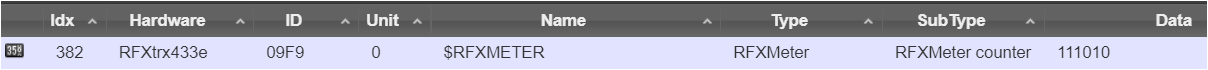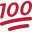# B4R Question[SOLVED] Convert Byte Array to String to Long

#### rwblinn

##### Well-Known Member
Longtime User
For a project exploring sending data from an Arduino with 433RF transmitter to Domoticz Homeautomation.
Created a B4R library rX10RF (wrapped and enhanced from X10RF lib) which enables to sent a Long (with max value FFFFFF, 3 Bytes) to a RFXMeter in Domoticz.
One of the tests is to sent the state 0 or 1 of various switches as Long. As the rX10RF library Long uses 3 Bytes, 3 switches can be defined, i.e. 010001 (switch 1=1, 2=0, 3=1).

Instead using Bytes, thinking of creating a Long value from the switch states.
Example: 5 switches, with state 0,1,1,0,1, converted to Long with leading 1 = 101101. The Long 101101 is then parsed by Domoticz (split into array with 1+5 entries).

Question: How to create the Long 101101 from the Byte array?

Start snippet but got stuck...
B4X:
``````'Byte Array for the 5 switches which have state 0 or 1
Private switches(5) As Byte
'Set random state for the switches. Use larger random range instead 0,1 to get higher probability for value changes.
For i = 0 To 4
switches(i) = IIf(Rnd(0,10) <= 5, 0, 1)
Next
' Loop over the Byte array to check the values
For Each b As Byte In switches
Log("b=", b, " (", bc.HexFromBytes(Array As Byte(b)), ")")
'b=1 (01) ...
Next
'Create Long i.e. 101101 from the Byte array
'???``````

Last edited:
Solution
not the best way probably
using decimal digits as binary digits is mildly inefficient, ie you're only using 2 digit values (0 and 1) out of a possible 10 digit values (0, 1, 2, ,3, 4, 5 ,6 ,7, 8 and 9) but if you're doing it that way because it's easier to read at the other end, then: fair enough, no worries3 bytes = 24 bits = values 0 to 16777215 = enough room for your leading 1 followed by up to 7 switch values of 0 or 1

(or even 7 eg rotary selectors or multi-level dimmers each having up to 7 states ie 0, 1, 2, 3, 4, 5 and 6)

Rather than using strings (because this), consider just building the Long value directly, eg with these three lines:

B4X:
``````'Switches state string starting with leading 1
Dim switchesState...``````

#### emexes

##### Expert
Done in B4J not B4R but should all still work except may for the string concatenations in the Log()s

Pack and unpack switch array to/from integer value:
``````Dim bc As ByteConverter

Dim NumSwitches As Int = 5

Dim Switches(NumSwitches) As Byte    'or any integer type

'Byte Array for the 5 switches which have state 0 or 1
'Set random state for the switches. Use larger random range instead 0,1 to get higher probability for value changes.
For i = 0 To NumSwitches - 1
switches(i) = Rnd(0, 2)
Next

' Loop over the Byte array to check the values
For Each b As Byte In switches
Log("b=" & b & " (" & bc.HexFromBytes(Array As Byte(b)) & ")")
'b=1 (01) ...
Next

'Create Long i.e. 101101 from the Byte array
Dim AllSwitches As Int = 0    'or any integer type big enough to hold value of 2 ^ NumSwitches - 1
Dim OneBit As Int = 1
For I = 0 To NumSwitches - 1
If switches(i) <> 0 Then
AllSwitches = AllSwitches + OneBit    'poor man's bitwise or
End If

OneBit = OneBit + OneBit    'poor man's shift left to next bit column value
Next

Log("All switches in one number = " & AllSwitches & " (decimal) or " & bc.HexFromBytes(bc.IntsToBytes(Array As Int(AllSwitches))) & " (hexadecimal)")

'now to unpack individual switch values from bits packed in single integer

Dim Switchback(NumSwitches) As Byte    'or any integer type

Dim OneBit As Int = 1
For I = 0 To NumSwitches - 1
If Bit.And(AllSwitches, OneBit) <> 0 Then
Switchback(I) = 1
End If
OneBit = OneBit + OneBit
Next

For I = 0 To NumSwitches - 1
Log("Switchback(" & I & ") = " & Switchback(i))
Next``````

Log output::
``````Waiting for debugger to connect...
Program started.
b=1 (01)
b=1 (01)
b=0 (00)
b=1 (01)
b=0 (00)
All switches in one number = 11 (decimal) or 0000000B (hexadecimal)
Switchback(0) = 1
Switchback(1) = 1
Switchback(2) = 0
Switchback(3) = 1
Switchback(4) = 0``````

#### emexes

##### Expert
TBH if you've only got a small number of switches, you might be better doing the packing and unpacking "manually" eg:

B4X:
``````Dim NumSwitches As Int = 5
Dim Switches(NumSwitches) As Byte
Switches(0) = Rnd(0, 2)
Switches(1) = Rnd(0, 2)
Switches(2) = Rnd(0, 2)
Switches(3) = Rnd(0, 2)
Switches(4) = Rnd(0, 2)

'pack Switches() to AllSwitches

Dim AllSwitches As Int = 0    'or byte, for up to 7 switches
If Switches(0) <> 0 Then AllSwitches = AllSwitches + 1
If Switches(1) <> 0 Then AllSwitches = AllSwitches + 2
If Switches(2) <> 0 Then AllSwitches = AllSwitches + 4
If Switches(3) <> 0 Then AllSwitches = AllSwitches + 8
If Switches(4) <> 0 Then AllSwitches = AllSwitches + 16

'unpack AllSwitches to Switches()

Dim Switches(NumSwitches) As Byte    'to clear all back to 0'
If Bit.And(AllSwitches,  1) <> 0 Then Switches(0) = 1
If Bit.And(AllSwitches,  2) <> 0 Then Switches(1) = 1
If Bit.And(AllSwitches,  4) <> 0 Then Switches(2) = 1
If Bit.And(AllSwitches,  8) <> 0 Then Switches(3) = 1
If Bit.And(AllSwitches, 16) <> 0 Then Switches(4) = 1``````

Last edited:

#### rwblinn

##### Well-Known Member
Longtime User
@emexes: Thanks for the steer.
Took another approach using JoinStrings to get the Long in kind of binary format = not the best way probably.
Will explore if can use Bit.And instead of JoinStrings and also check the Long length within the 3 Byte limitation.

B4X:
``````'Switches state string starting with leading 1
Dim switchesState As String = "1"
'Add the switch state 0 or 1 to the switchesState string
For i = 0 To NumSwitches - 1
Dim n As Byte = IIf(Rnd(0,10) <= 5, 0, 1)
switchesState = JoinStrings(Array As String(switchesState, n))
Log("Switch ", i, "=", n)
Next
Dim switchesStateValue As Long = switchesState
Log("Sending to Domoticz ", NumSwitches, " switches with state packed as Long ", switchesStateValue, " (with leading 1)")

'Switch 0=1
'Switch 1=1
'Switch 2=0
'Switch 3=1
'Switch 4=0
'Sending To Domoticz 5 Switches with state packed As Long 111010 (with leading 1)``````

Here screenshot Domoticz Device with Long received. An automation event (Lua) parses the data and sets the switches state indicators.Last edited:

#### emexes

##### Expert
not the best way probably
using decimal digits as binary digits is mildly inefficient, ie you're only using 2 digit values (0 and 1) out of a possible 10 digit values (0, 1, 2, ,3, 4, 5 ,6 ,7, 8 and 9) but if you're doing it that way because it's easier to read at the other end, then: fair enough, no worries3 bytes = 24 bits = values 0 to 16777215 = enough room for your leading 1 followed by up to 7 switch values of 0 or 1

(or even 7 eg rotary selectors or multi-level dimmers each having up to 7 states ie 0, 1, 2, 3, 4, 5 and 6)

Rather than using strings (because this), consider just building the Long value directly, eg with these three lines:

B4X:
``````'Switches state string starting with leading 1
Dim switchesState As String = "1"
Dim FasterSwitchesStateValue As Long = 1
'Add the switch state 0 or 1 to the switchesState string
For i = 0 To NumSwitches - 1
Dim n As Byte = IIf(Rnd(0,10) <= 5, 0, 1)    'just realized this is 60:40 not 50:50
switchesState = JoinStrings(Array As String(switchesState, n))
FasterSwitchesStateValue = FasterSwitchesStateValue * 10 + n    'changing 10 to 2 will pack in binary (base 2) rather than decimal (base 10) = 3x as many switches
Log("Switch ", i, "=", n)
Next
Dim switchesStateValue As Long = switchesState
Log("Sending to Domoticz ", NumSwitches, " switches with state packed as Long ", switchesStateValue, " (with leading 1)")
Log("Sending to Domoticz ", NumSwitches, " switches with state packed as Long ", FasterSwitchesStateValue, " (with leading 1)")

'Switch 0=1
'Switch 1=1
'Switch 2=0
'Switch 3=1
'Switch 4=0
'Sending To Domoticz 5 Switches with state packed As Long 111010 (with leading 1)``````

and once you've confirmed that the integer math method works, then the string method lines 2, 7, 11 and 12 can be removed / commented out.

Last edited:
••Johan Hormaza and rwblinn

#### rwblinn

##### Well-Known Member
Longtime User
FasterSwitchesStateValue = FasterSwitchesStateValue * 10 + n 'changing 10 to 2 will pack in binary (base 2) rather than decimal (base 10) = 3x as many switches

YES, this simple math is what I have been looking for (could not get my head around). Working fine.
Thanks again for the help and learning.
Now up to next X10RF sample solutions...

•emexes

Replies
3
Views
946
Replies
6
Views
1K
B4R Tutorial Strings and Bytes
Replies
8
Views
22K